# There

There are 32 trees of three species in the orchard. 25% cherries, three eighty pears, others plums. How many plums are in the orchard?

s =  12

### Step-by-step explanation:

32 = 25/100•32 + 3/8 • 32 + s

200s = 2400

s = 12

Our simple equation calculator calculates it.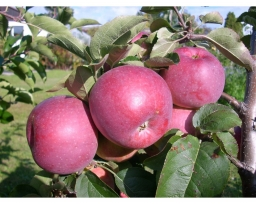Did you find an error or inaccuracy? Feel free to write us. Thank you!Tips to related online calculators
Need help to calculate sum, simplify or multiply fractions? Try our fraction calculator.
Do you have a linear equation or system of equations and looking for its solution? Or do you have a quadratic equation?

## Related math problems and questions:

• Trees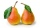Two-fifths of the trees in the orchard are apple trees, one-sixth a pear. The remaining 26 trees are cherries. How many pears are in the orchard?
• Orchard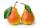Three-fifths of the orchard are apple trees, one-third of the trees are cherries. The remaining 5 trees are pears. How many trees are in the orchard?
• In the orchardIn the orchard, they planted 25 apple trees, 20 pears, 15 plums and 40 marbles. A strong late frost, however, destroyed a fifth of all new trees. Unfortunately, it was all the trees of one kind of fruit. What is the probability that the plums have died ou
• Trees - orchardThe 3/5 trees are apple trees and 1/3 of the trees are cherries. The remaining 5 trees are pear trees. How many trees are in the orchard.
• Orchard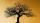3/5 of the trees in the orchard are apples, 1/3 are cherries and the remaining five trees are pear. How many trees are in the orchard?
• In the cinema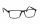There were 560 people in the cinema. 35% of them wore glasses. Of this number, 25% were women. How many men wore glasses?
• TreesFrom the total number of trees in the orchard, there are two-fifths pearls and apples are three eighty. The rest of the trees are 9 ceremonial. How many trees are in the set?
• Prospering studentsThere are girls and 30 boys in the class. Boys prospering 28, girls all. How many girls are in the class and how many boys are there, if all the prospering students are 95%?
• Cut trees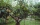There are 1,200 trees in the grove, of which 55 percent are deciduous, the remaining coniferous. Workers cut down 35 percent a) trees, b) deciduous trees, c) coniferous trees. How many trees now have this grove?
• TreesIn the orchard, 38 apple trees and 20 pear trees grew. How many trees there grow?
• Cinema ticketsCinema sold 180 tickets this Thursday, which is 20%. Monday 14%, Tuesday 6%, Wednesday 9%, Friday 24%, Saturday 12%, and Sunday 15%. How many tickets were sold per week?
• An orchard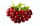In an orchard, there are 28 apple trees, 27 orange trees, and 14 cherry trees. What is the ratio of apple trees to cherry trees?
• Stock market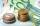Simon one day decided to invest € 62000 to the stock market. After six months, he invested July 25 stock markets fell by 47%. Fortunately for Simon, from July 25 5 to October 25 his shares have risen by 39%. Simon is then: ...
• In the classroomThere are 30 boys and a few girls in the class. In the six months, 28 boys and all girls benefited, which was 95% of all pupils. How many pupils are there in the classroom?
• The number 72The number 72 increase by 25%. By how much % will you have to reduce the number you created to get the number 72 again?
• Final examThere are 5 learners in a class that has written a final exam Aleta scored 55% vera scored 36% and Sibusiso scored 88% if Thoko scored 71% and the class average was 63%. What was Davids score as a percentage?
• Two math problems1) The sum of twice a number and -6 is nine more than the opposite of that number. Find the number. 2) A collection of 27 coins, all nickels, and dimes, is worth \$2.10. How many of each coin are there? The dime, in United States usage, is a ten-cent coin.